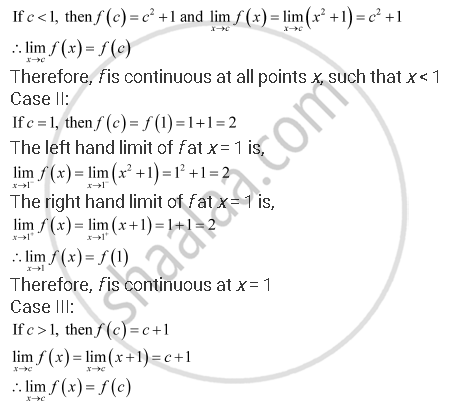Share

# Find All Points of Discontinuity of F, Where F is Defined by F(X) = {(X+1, If X >= 1),(Xsqrt2 + 1,If X < 1) - CBSE (Commerce) Class 12 - Mathematics

#### Question

Find all points of discontinuity of f, where f is defined by

f(x) = {(x+1, "," if x >= 1),(x^2 + 1, ","if x < 1):}

#### Solution

The given function f is f(x) = {(x+1, "," if x >= 1),(x^2 + 1, ","if x < 1):}

The given function f is defined at all the points of the real line.

Let c be a point on the real line.

Case I:Therefore, f is continuous at all points x, such that x > 1

Hence, the given function has no point of discontinuity.

Is there an error in this question or solution?

#### APPEARS IN

NCERT Solution for Mathematics Textbook for Class 12 (2018 to Current)
Chapter 5: Continuity and Differentiability
Q: 10 | Page no. 159

#### Video TutorialsVIEW ALL 

Solution Find All Points of Discontinuity of F, Where F is Defined by F(X) = {(X+1, If X >= 1),(Xsqrt2 + 1,If X < 1) Concept: Concept of Continuity.
S